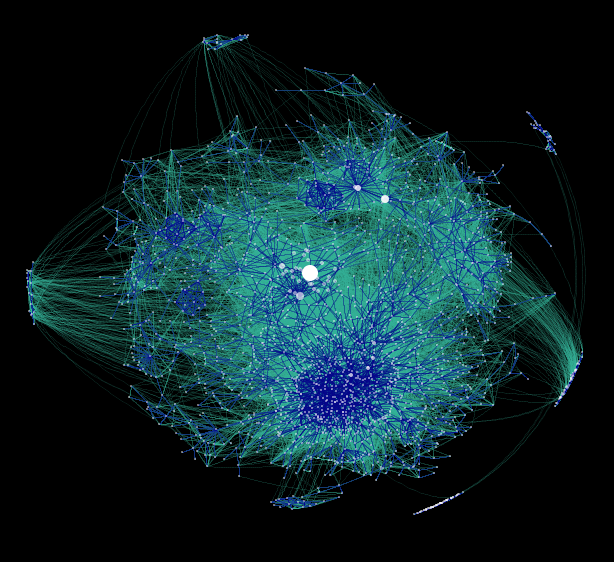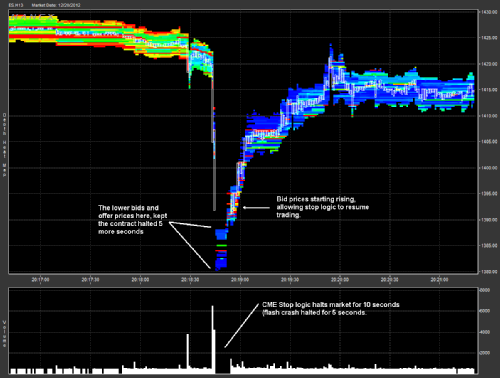# Studying Probability and Statistics

Probability and Statistics spans a range from pure mathematical analysis, through the modelling of random processes, to heavily computational applied statistics such as data mining. It is of interest both as an academic subject and because of its immense importance in applications. Many of the careers followed by mathematical sciences graduates involve probabilistic modelling or sophisticated data analysis. In fact, Hal Varian, Google’s Chief Economist has recently said, "I keep saying the sexy job in the next ten years will be to be a statistician." See Dream job of the next decade.A graph showing how part of the blogosphere is connected. Modelling and analysing these sort of random structures has become an important part of modern Statistics.

Warwick's research group in probability and statistics is one of the largest in Britain. Its staff and its lecture courses are spread between the Mathematics and Statistics Departments. Here is how to get the best out of what is available.

Course structure

Year 1. (For 2022/23 entry and later)

Higher level modules build on the linear algebra, analysis and probability studied in first year. The Year 1 core module ST120 Introduction to Probability is required for most of the higher level probability.

ST121 Statistical Laboratory is an optional module where you can gain experience of simulation and data analysis with the widely used statistical programming language R (and R studio). Several later statistics modules will assume that students know how to use R. For example, courseworks will often requires it to do meaningful analysis on data sets.

For students commencing their studies in or before 2021/22, the relevant year 1 modules are ST111, ST112 and ST104.

Year 2.

If you want to have the option of taking third year Statistics modules, then it is important to take ST220 Introduction to Mathematical Statistics (or ST232 Introduction to Mathematical Statistics for 2022/23 entry). In this module you study statistical inference, the process of drawing conclusions from data that is subject to random variation, and the mathematics it is based upon.

The module ST202 Stochastic processes (or ST227 Stochastic Processes for 2022/23 entry) introduces the Markov property and its use in building probabilistic models for diverse applications such as random networks, population growth, queues and epidemics.

The module ST221 Linear Statistical Modelling (or ST231 Linear Statistical Modelling with R) covers ideas and methods of statistical modelling and statistical model exploration. The module also introduces students to the application of R software (and R studio) and its use as a professional tool for statistical modelling, specifically for working with linear models in a variety of different scenarios.

Mathematics students wishing to study ST221 Linear Statistical Modelling must take this module in Year 2, it is not available in Year 3 (even as an unusual option).

Mathematics students interested in transferring to Year 3 of the Mathematics and Statistics programme, must take Introduction to Mathematical Statistics, Stochastic Processes and Linear Statistical Models.

Year 3 and beyond.... (See Note 1)

Year 3 students that did not take Introduction to Mathematical Statistics have the opportunity to study this module in Year 3, but you must register for the Year 3 variant.

Where third year Statistics modules state that ST218 Mathematics Statistics Part A and ST219 Mathematics Statistics B are pre-requisites, mathematics students should consider ST220/ST226/ST232 as equivalent.

Third year Statistics modules cover a wide range of methods and applications showing how mathematics can be used to extract information from data and make decisions. These modules include Multivariate Statistics, Bayesian Statistics, Medical Statistics, Designed Experiments, and Bayesian Forecasting and Intervention.

In the modules Random discrete Structures, Markov processes and Percolation Theory and Applied Stochastic Processes you can study various more sophisticated mathematical models for random structures and processes that are both intrinsically interesting mathematical objects and also very important for applications.A "flash crash" caused by high frequency trading on the stock market. Hedge funds make extensive use of probability theory to decide when to buy and sell financial contracts. The basic principles are covered in module Introduction to Mathematical Finance.

Advanced probability requires a mathematical framework called measure theory developed by some of the greatest mathematicians of the twentieth century. It is taught at Warwick in Probability Theory and Measure Theory. Often students who are strong in analysis find that they enjoy studying measure-theoretic probability. This leads to advanced modules such as Brownian Motion and Stochastic Analysis. These are useful for students who wish to work as financial analysts in the City, work in scientific research and development, or who are considering continuing on to do a PhD in Mathematics or Statistics.

Note 1. Names are indicative of the content and may not reflect the actual module name.

Course transfers to Data Science, Mathematics and Statistics or MORSE

If you wish to transfer to Data Science, Mathematics and Statistics or MORSE at the end of Year 1 or Year 2, then you must meet the course transfer requirements available in the Department of Statistics handbook for external students.